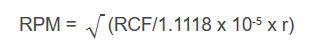# RPM and G-Force: Which Is More Important and Why?

By Aimee O'Driscoll, 28 March 2019

There is often confusion about the relationship between RPM and RCF and which parameter is most important in centrifugal applications. Below, we explain both terms, discuss which measure is more important, and provide the formula to convert between the two.

RPM (revolutions per minute) is the way in which we describe how fast a centrifuge is going. This is the rate at which the rotor is revolving regardless of its size.

G-Force or RCF (relative centrifugal force) is the force being exerted on the rotor contents. RCF is a result of the rotor revolving so is dependent on RPM.The key attributes for the Z306 Universal Centrifuge show the max RPM and RCF for this unit.

In a centrifugal process, RCF is what is affecting your samples, so it’s important that this is known. RPM is less helpful (unless rotors are known to be the same size), although it can be used to calculate the required RCF. In this case, you also need to know the radius of the rotor.

Note that most rotors cannot operate at a centrifuge's max RCF, and users should be aware of the RPM/RCF limits of any particular rotor when considering a rotor or centrifuge purchase.

To calculate RCF from RPM, you can use the following equation:Where r = radius of the rotor

Rearranging the equation will enable you to calculate the RPM required for a given RCF, for example, if you’re transferring a process to new equipment.

To calculate RPM from RCF, use the following equation:Where r = radius of the rotor

If you’d rather not do the calculations yourself, there are online converters available to help, such as the one offered by In silico.